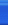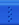# Impossible is Nothing

Impossible is NothingIT博客首页新随笔联系聚合管理好久没有写日记了，呵呵最近挺忙论文工作，愁死了。

今天小师妹问了我一个问题，关于sizeof，居然没有解答出来，悲哀，呵呵。进行了一顿狂补。结果如下

1。 问题

class     A
{
int     a;
public:
op(){a=14;};
int     as     (){return     a*a;}
};
与     class     B
{
int     a;
public:
op(){a=14;};
};
大小一样
编译器究竟把成员函数放在哪里？成员函数又怎么样和类关联起来？

解答如下：

class     A
{
int     a;
public:
op(int   value){a=value;};
public
virtual   int   as(){return   a*a;}
};

对于以上的类的说明，当创建一个实例时，其内存结构如下(不同的C++实现略有不同)：
+0000:   成员变量   int   a
+0004:   \$A\$vtable指针     -->         +0000:   成员函数指针   as

当C++编译以上的类时，它会产生类似以下的成员函数(不同的C++实现略有不同)：
//op函数
int   \$A\$op@i_(A*   this,   int   value)   {this->a   =   value;}
//as函数
int   \$A\$as@_(A*   this)   {return   this->a   *   this->a;}

也就是说，函数名本身就指明了函数是属于哪个类的(连参数类型都有)，因此，编译器在编译代码时，可以直接调用该类的成员函数，而不需要类本身提供任何信息。
例(0):
A   a;
对于函数调用：
此时，a是A的实例，因此编译生成代码为：

例(1)：
A   a;
对于函数调用：
a.as();
此时，a是A的实例，因此编译生成代码为：
\$A\$as@_(&a);

例(2)
A*   pa;
对于函数调用：
对应生成代码为：

例(3)
A*   pa;
对于函数调用：
pa->as();
对应生成代码为：
(pa->\$A\$vtable)(pa);

请注意：
如果不是用指针调用，那么是不是virtual函数，调用方法是一样的，参见例(0)和例(1)
如果是指针调用，例(2)和例(3)就是调用virtual函数和普通成员函数的区别。就因为有这区别，所以virtual函数必须在类中占一席之地

2。关于sizeof大小的问题 （可参考http://zhang4331.blog.com.cn/archives/2007/2509985.shtml

class A {
public:
virtual void fun0() { cout << "A::fun0" << endl; }
int a;
int b;
};
int main(int argc, char* argv[])
{
A  a;
cout << "Size of A = " << sizeof(a) << endl;
return 0;
}

class A {
public:
virtual void fun0() { cout << "A::fun0" << endl; }
int a;
int b;

double c；
};
int main(int argc, char* argv[])
{
A  a;
cout << "Size of A = " << sizeof(a) << endl;
return 0;
}
结果为24。double+Two int＋Virtual（原为四）＝8+8+8＝24

3。单继承与多继承

#include "iostream.h"
#include "string.h"
#include "stdlib.h"

class A {
public:
virtual void f() { cout << "A::f" << endl; }
};
class B :public A{
public:
virtual void f() { cout << "B::f" << endl;}
};
class C :public A {
public:
virtual void f() { cout << "C::f" << endl;}
};
class Drive : public C {
public:
virtual void f() { cout << "D::f" << endl;}
};

int main(int argc, char* argv[])
{
A a;
B b;
C c;
Drive d;
cout << sizeof(a) << endl;
cout << sizeof(b)  << endl;
cout << sizeof(c) << endl;
cout << sizeof(d)  << endl;
system("pause");
return 0;
}class A {public: virtual void f() { }};

class B {public: virtual void f() { }};

class C {public: virtual void f() { }};

class Drive : public A, public B, public C {};

int main()

{ Drive d;

cout << "Size is = " << sizeof(d) << endl;

return 0;}4。但是在class A{}中sizeof（A）却为1，不知道为什么？

class B2
{
void fun(void);
}

B2 b2;
int a;

posted on 2008-04-26 10:22 痛并快乐着 阅读(516) 评论(0)  编辑 收藏 引用 所属分类: Unix程序